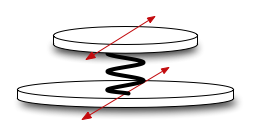ModalysObjects > Object Reference > Two-Mass - Mono-directional
page précédentepage suivante

# Two-Mass - Mono-directional

Description

A simple bi-directional two-mass model that can serve as a hammer, plectrum, finger, etc. Generally the two-mass model has a small mass and a large mass; the small one will be "connected" to the object being excited, and the large one will be manipulated by the user. This is similar to the bi-two-mass, except that it only vibrates in the trans0 direction. It is therefore more computationally efficient for single-direction interactions like plucking and striking objects.## (make-object 'mono-two-mass ... )

Lisp Syntax and Default Values

A 'mono-two-mass can be created using the following Lisp syntax (the default values are shown for each of the physical parameters):

(make-object 'mono-two-mass

(small-mass .01)

(large-mass .01)

(stiffness0 15000)

(freq-loss0 100)

(const-loss0 0))

Parameters

All physical parameters can be numerical values or controllers.

• small-mass: The mass of the small mass in kg.
• large-mass: The mass of the large mass in kg.
• stiffness0: The stiffness of the spring in the 'trans0 (horizontal) direction.
• freq-loss0: The usual frequency loss parameter. See the General Object Information for more info.
• const-loss0: The usual constant loss parameter. See the General Object Information for more info..

As always, the term horizontal used above is provided for simplification.

Accesses

A 'bi-two-mass can be accessed only in its sole vibrational direction: trans0.

(make-access my-mono-two-mass my-controller 'trans0)

If the value of my-controller is 1, the access is on the small mass. If my-controller is 0, the access is on the large mass. (A value in-between takes a weighted combination of the two points.)

## Options

There are no special options for the mono-two-mass.

page précédentepage suivante